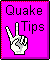Sudden Stop

The worst case situation should prepare for an impact after being dashed equivalent to a drop of 500 feet. This presumes no protections around the object or person to prevent impact injury.
ZetaTalk

What does a 500 ft drop mean? What does padding and no padding mean? A 500 ft drop will cause a G-force that has me deeply concerned. The following analysis will show you why. I have been going around and around with it over the last few weeks trying to find an error. I hope someone can find an error somewhere in the math or logic. If we first assume terminal velocity is not reached and air viscosity has no slowing effect. If we now assumed there is enough padding to allow for 6" of motion before the item completely squashes the padding and assuming the padding decelerates the object uniformly throughout the 6" of motion. Note: A typical mattress is say 6" to 8" thick and with someone laying on it squashes a bit so that in the best of conditions 4" to 6" of motion for deceleration is reasonable.

V = (2gS)^.5 = Velocity due to free fall of gravity
v = (2as)^.5 = velocity at start of deceleration
Set V = v and solve for "a". This becomes:
a = (S/s)g = (S/s)G = Deceleration in "G-force".
g = gravitational constant 32 ft/sec^2 = 1 G.
S = 500 ft distance of free fall.
s = Deceleration or stopping distance of 6" = .5 ft.
a = (500ft/.5ft)G = 1000 G of force.

Note: The table below was determine from this formula using different distances of deceleration. Assuming no air friction. How fast would an object be going, if dropped from a height of 500 ft?

v = (2gs)^.5 = ((2*32 ft/sec^2)*500 ft)^.5 = 179 ft/sec
= 179 ft/sec*1mile/5280ft*3600sec/hr = 122 miles/hr.
Note: (1 ft/sec = .682 miles/hr)

What is free fall terminal velocity or maximum speed? A sky diver friend of mine tells me this is from 120 miles/Hr (body horizontal) to over 200 miles/Hr (body vertical). The above is within the 120 to 200 mile/Hr range so that for our purposes we are probably safe assuming no air resistance in determining maximum speed of fall. Stopping "s" distance and amount of G-force experienced:

A 500 ft drop results in a relative speed of 122 MPH
A .5" deceleration distance results in 12,000 G-force
A 1" deceleration distance results in 6,000 G-force
A 4" deceleration distance results in 1,666 G-force
A 6" deceleration distance results in 1,000 G-force
A 1 ft deceleration distance results in 500 G-force
A 2 ft deceleration distance results in 250 G-force
A 10 ft deceleration distance results in 50 G-force
A 100 ft deceleration distance results in 5 G-force

Summary: For example, what this is saying is that if the proper padding is used so as to uniformly stop a fall from 500 ft in a 6" distance, that the body falling would experience a force of 1000 times it's weight as it stops. To put this in simple terms. Stack 1000 bodies your same weight on top of yours, put them on a typical mattress and we have two problems. Your body at the bottom is probably crushed with broken bones and the mattress went flat a long time ago, offering no padding. All this would happen rapidly depending on the padding thickness or stopping distance "s". Yet the G-forces will be there. Now if one wanted to experience less than 5 G-force then expect to use over a 100 ft of padding on all sides. This becomes absurdly ridiculous.

A 500 ft drop, with no padding at the bottom, is equivalent to a sky diver hitting the ground without a parachute. There have been sky divers falling at about 120 miles/Hr whose parachutes have not opened, and they have lived through it, with only some broken bones. Assuming the ground-body combination flexed 1" to 4" then the G-force could be between 6,000 G and 1,666 G. I don't know what the maximum limit of what a body can withstand and still live through it is. I do know, that for us average folks it's probably not as low a 9 G, but a lot lower than 1000 G. One needs to remember the duration of G-force is not going to be as long as tests done on individuals in centrifuges to simulate fighter pilots, or astronaut reentry situations.

There is the possibly of getting high G forces with low amplitude vibration of short duration's. Duration of G force is a big factor. High G force short duration is less destructive than the same G force for a longer duration. Longer duration will compress any padding and then transmit the acceleration to the component or body being padded. The short duration high G-force is expected and would be already shielded for, once the longer duration G-force shielding is in place. By defining must with stand a given drop height the duration is defined for each stopping distance, and thus becomes a good way to define the problem.

As far as electronic component protection: 4" to 1 ft. of padding would provide protection such that each component of the electronic unit would experience no less than 1666 to 500 times it's own weight in trying to tare the unit apart. The overall effect of all component motion is to attempt to squash the unit flat. Notes: One should note, that padding does not squish to nothing. It will have a squashed thickness that is not a part of the "s-distance" above. Also, padding being squashed in the real world will not produce a uniform deceleration. Expect the deceleration G-force to increase, to some degree, the more compressed the padding.

Offered by Mike.